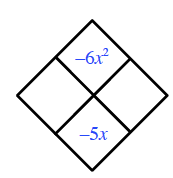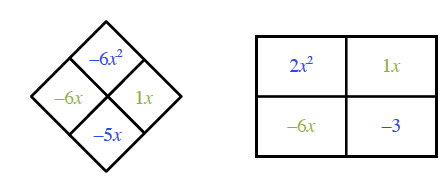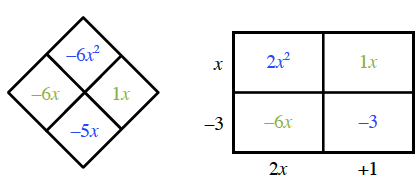### Home > INT3 > Chapter Ch12 > Lesson 12.2.1 > Problem12-86

12-86.

Factor and simplify each expression below. Homework Help ✎

1. $\frac { x ^ { 2 } - 4 } { x ^ { 2 } + 4 x + 4 }$

Factor and then reduce.

2. $\frac { 2 x ^ { 2 } - 5 x - 3 } { 4 x ^ { 2 } + 4 x + 1 }$

For the numerator, what numbers multiply to $−6$ and add to $−5$?

Put these numbers in a generic rectangle (these create your "middle term") along with the first and last terms.
Now find the greatest common factors of each row and column.

See the generic rectangle.
$2x^{2} − 5x − 3 = \left(x − 3\right)\left(2x + 1\right)$

Now factor the denominator and simplify.